### Paper 2 - Maths

Question 1: For a complex number z, let Re(𝑧) denote the real part of z. Let S be the set of all complex numbers z satisfying 𝑧4 − |𝑧|4 = 4 𝑖𝑧2, where i = √(-1) . Then the minimum possible value of |𝑧1 −𝑧2|2, where 𝑧1, 𝑧2 ∈ 𝑆 with Re(𝑧1) > 0 and Re(𝑧2) < 0, is _____

Solution:

Solution:

z4 - |z|4 = 4iz2

z4 - 2|z|2|bar z|2 = 4iz2

Because, z(bar z) = |z|2

*here bar z is conjugate of z

Z2 = 0 or z2 – (bar z)2 = 4i

Let z = x + iy

We know

z2 – (bar z )2 = 4i

(x + iy)2 – (x – iy)2 = 4i

4ixy = 4i

xy = 1

Now (z1– z2)2 = (x1– x2)2 + (y1– y2) 2

= x12 + x22 + y12 + y22– 2x1x2– 2y1 y2

= x12 + x22 + y12 + y22 + 2x1(-x2 ) + 2y1 (-y2)

Now A.M. ≥ G.M.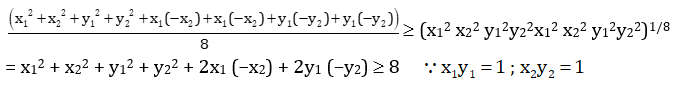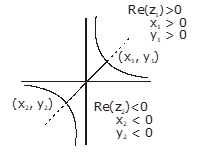Question 2: The probability that a missile hits a target successfully is 0.75. In order to destroy the target completely, at least three successful hits are required. Then the minimum number of missiles that have to be fired so that the probability of completely destroying the target is NOT less than 0.95, is_____.

Solution:

Solution:

Given, P(Hit) = 0.75 = 3/4 = P(H)

and P(Hit not) = 0.25 = (1/4) P(bar H)

P(target Hit) ≥ 0.95

1 - P(target not hit in n throws) ≥ 0.95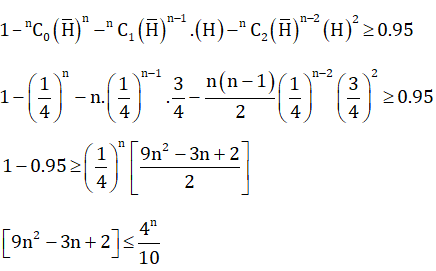Now, check n = 6

Question 3: Let O be the centre of the circle x2 + y2 = r2, where r > √5/2 . Suppose PQ is a chord of this circle and the equation of the line passing through 𝑃 and 𝑄 is 2x + 4𝑦 = 5. If the centre of the circumcircle of the triangle 𝑂𝑃𝑄 lies on the line 𝑥 + 2𝑦 = 4, then the value of r is_____

Solution:

Solution:

Given,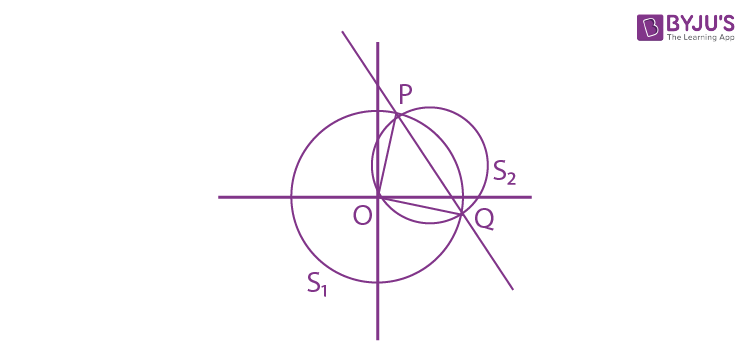S1: x2 + y2 = r2 where r > √5/2

C1 = (0,0)

Now let S2: x2 + y2 + ax + by = 0

C2 = (-a/2, -b/2)

RA of S1 = 0 & S2 = 0 is PQ

PQ: S1 – S2 = 0

PQ: ax + by + r2 = 0 ………………… (1)

Given PQ: 2x + 4y - 5 = 0 ………………… (2)

On comparing equation (1) & (2)

(a/2) = (b/4) = (r2/-5)…………..…..(3)

Also, centre of S2 lies on x + 2y = 4

(-a/2) – b = 4 …(4)

From equation (3) & (4)

r = 2

Question 4: The trace of a square matrix is defined to be the sum of its diagonal entries. If A is a 2 x 2 matrix such that the trace of A is 3 and the trace of 𝐴3 is −18, then the value of the determinant of A is_____

Solution:

Solution:

Let A =

$$\begin{bmatrix} a & b\\ c& d \end{bmatrix}$$
…(1)

Tr(A) = 3 (Given)

a + d = 3

d = 3 – a put in equation (1)

A =

$$\begin{bmatrix} a & b\\ c& 3-a \end{bmatrix}$$

Tr(A3) = -18 (Given)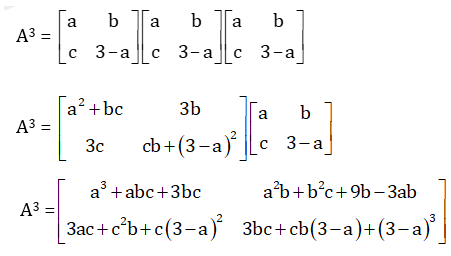Tr (A3) = a3 + abc + 3bc + 3bc + 3bc - abc + (3 - a) 3= - 18

a3 + 9bc + (3 -a) 3 = -18

a3 + 9bc + 27 – a3 - 3.3a (3 - a)= - 18

a2 - 3a + bc = -5 ……………… (2)

Now |A| = a(3 – a) – bc

= 3a – a2 – bc = 5 (From equation (2))

|A| = 5

Question 5: Let the functions f: (−1,1) → R and g: (−1, 1) → (−1, 1) be defined by f(x) = |2x − 1| + |2x + 1|and g (x) = x − [x], where [x] denotes the greatest integer less than or equal to x. Let fog: (−1,1) → R be the composite function defined by (fog)(x) = f(g(x)). Suppose C is the number of points in the interval (−1, 1) at which fog is NOT continuous, and suppose d is the number of points in the interval (−1,1) at which fog is NOT differentiable. Then the value of c + d is _____

Solution:

Solution:

f(x) = |2x − 1| + |2x + 1|

f(x) = -4x at x ≤ -1/2

f(x) = 2 at (-1/2) < x < (1/2)

f(x) = 4x at x ≥ ½

g(x) = x [x] = {x}

Now,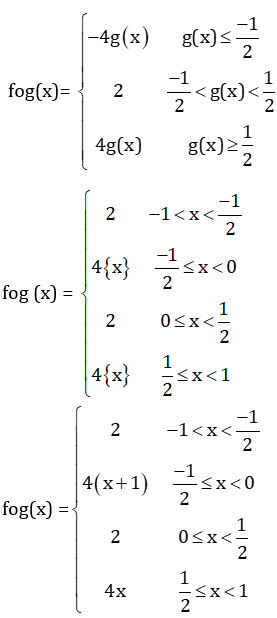Now check

fog is not continuous at x = 0 only => c = 1

fog is not differentiable at x = (-1/2), 0, (1/2), => d = 3

So, c + d = 4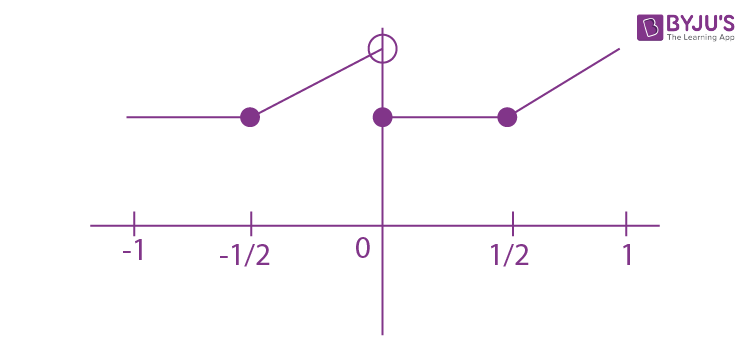Question 6: The value of the limit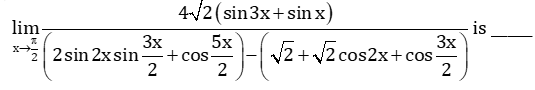Solution:

Solution:

Using transformation and submultiple angle formula in denominator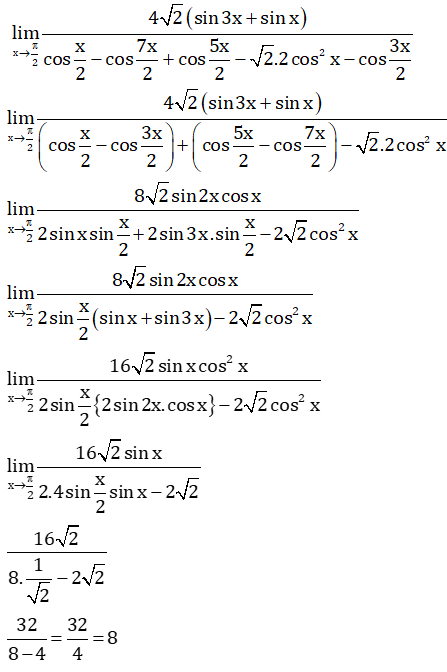Question 7: Let b be a nonzero real number. Suppose f = R → R is a differentiable function such that f(0) = 1. If the derivative f' of f satisfies the equation f’(x) = f(x)/(b2 + x2). For all x ∈ R, then which of the following statements is/are TRUE?

1. a) If b > 0, then f is an increasing function
2. b) If b < 0, then f is a decreasing function
3. c) f(x) f(−x) = 1 for all x ∈ R
4. d) f(x) − f(−x) = 0 for all x ∈ R

Solution:

Solution:

Given f’(x) = f(x)/(b2 +x2)

f’(x)/f(x) = 1/(b2 + x2)

On integrating both sides, we get

ln(f(x)) = (1/b) tan-1(x/b) + c

Put x = 0, so c = 0

Because f(0) = 1(Given)

a)

$$f(x) = e^{\frac{1}{b}tan^{-1}(\frac{x}{b})}$$

Because, f'(x) = f(x)/(b2 +x2)

f(x) is increasing function.

f(x) > 0 for all x ∈ R

c)

$$f(x) f(-x)= e^{(\frac{1}{b}tan^{-1}(\frac{x}{b}))-(\frac{1}{b}tan^{-1}(\frac{x}{b}))} = e^{0} = 1$$

d)

$$f(x) - f(-x)= e^{\frac{1}{b}tan^{-1}(\frac{x}{b})}-e^{\frac{1}{b}tan^{-1}(\frac{x}{b})} \neq \: 0\: \forall \: x \: \epsilon \: R$$

Question 8: Let a and b be positive real numbers such that a > 1 and b < 𝑎. Let P be a point in the first quadrant that lies on the hyperbola

$$\frac{x^2}{a^2} - \frac{y^2}{b^2} =1$$
. Suppose the tangent to the hyperbola at P passes through the point (1, 0), and suppose the normal to the hyperbola at P cuts off equal intercepts on the coordinate axes. Let Δ denote the area of the triangle formed by the tangent at P, the normal at P and the x-axis. If e denotes the eccentricity of the hyperbola, then which of the following statements is/are TRUE?

1. a) 1 < e < √2
2. b) √2 <𝑒< 2
3. c) Δ = a4
4. d) Δ = b4

Solution:

Solution:

Normal cuts equal intercepts

MN = –1

MT = 1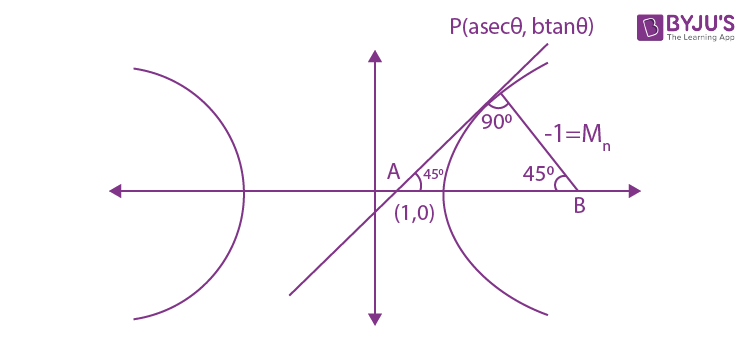Equation of tangent at P:

(x sec θ)/a – (y tan θ)/b = 1, if passes through (1, 0) then sec θ = a

MT = 1

+(b sec θ/a tan θ ) = 1

So, b = tan θ

b2 = a2 (e2 - 1)

e2 - 1 = sin2 θ

e2 = 1 + sin2 θ (Because 0 < θ < pi/2)

1 < e2 < 2

1 < e < √2

Area, Δ = (1/2)(AP) (AP) [because AP = BP]

= (1/2)[(1 – sec2θ )2 + (tan2θ )2] = tan4θ = b4

b = tanθ

Question 9: Let f: R → R and g: R → R be functions satisfying f(x + y) = f(x) + f(y) + f(x)f(y) and f(x) = xg(x)

For all x, y ∈ R. If limx->0 g(x) = 1, then which of the following statements is/are TRUE?

1. a) f is differentiable at every x ∈ R
2. b) If g(0) = 1,then g is differentiable at every x ∈ R
3. c) The derivative 𝑓′(1) is equal to 1
4. d) The derivative 𝑓′(0) is equal to 1

Solution:

Solution:

(x + y) = f(x) + f(y) + f(x)f(y) …(1) and

f(x) = xg(x) …(2)

Differentiating equation (1) with respect to y

f'(x + y).1 = f'(y) + f(x) f'(y) ....(3)

Put y = 0 in equation (3)

f'(x) = f'(0) + f(x)f'(0) ...(4)

From equation (2)

f(x) = xg(x)

differentiating with respect to x

f'(x) = xg'(x) + g(x) ....(5)

put x = 0 in equation (5)

we get f'(0) = g(0) = 1 (given)

f'(0) = 1 put in equation (4)

f'(x) = f(x) + 1

f'(x)/[f(x) + 1] = 1

On integrating both sides, we get

ln(f(x) + 1) = x + c …(6)

Put x = 0 in equation (6)

ln (1 + f(0)) = c ⇒ c = 0 put in equation (6)

1 + f(x) = ex

f(x) = ex – 1

f(1) = e – 1

f'(1) = f(1) + 1 = e - 1 + 1 = e, as f'(x) = f(x) + 1

f'(0) = f(0) + 1 = 0 + 1 = 1

g(x) = f(x)/x = [ex – 1]/x

We have check differentiability at x = 0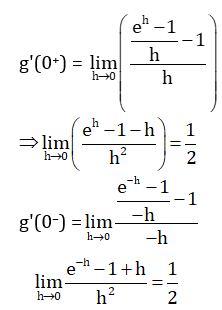Question 10: Let α, β, γ, δ be real numbers such that α 2 + β 2 + γ 2 ≠ 0 and α + γ = 1. Suppose the point (3, 2, −1) is the mirror image of the point (1, 0, −1) with respect to the plane α x + β y + γ z = δ. Then which of the following statements is/are TRUE?

1. a) α + β = 2
2. b) δ - γ = 3
3. c) δ + β = 4
4. d) α + β + γ = δ

Solution:

Solution:

Given equation of plane is α x + β y + γ z = δ

Q point is mid point of pp' = ((1+3)/2, (0+2)/2, (-1-1)/2) = (2, 1, -1)

Since point Q lie on the plane α x + β y + γ z = δ

α (2) + β (1) + γ (-1) = δ …(1)

pp’ is normal to given plane

α/2 = β/2 = γ/0 = λ (let)

α = 2 λ, β = 2λ , γ = 0

Because α + γ = 1 (given)

2 λ + 0 = 1

λ = ½

Therefore, α = 2 λ = 2 x ½ = 1

β = 2λ = 1 and γ = 0

Now, putting all values of α, β and γ in equation (1), we get

δ = 3

Therefore, δ - γ = 3

δ + β = 3+1 = 4

α + β + γ = 1+1+0 = 2 (≠ δ )

α + β = 1+1 = 2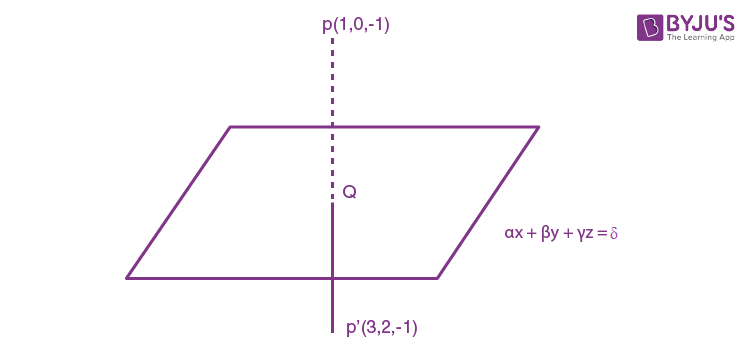Question 11: Let a and b be positive real numbers. Suppose

$$\vec{PQ} = a \hat{i} + b \hat{j}$$
and
$$\vec{PS} = a \hat{i} - b \hat{j}$$
are adjacent sides of a parallelogram PQRS. Let,
$$\vec{u}$$
and
$$\vec{v}$$
be the projection vectors of
$$\vec{w} = \hat{i} + \hat{j}$$
along
$$\vec{PQ}$$
and
$$\vec{PS}$$
, respectively. If
$$|\vec{u}| + |\vec{v}| = |\vec{w}|$$
and if the area of the parallelogram 𝑃𝑄𝑅𝑆 is 8, then which of the following statements is/are TRUE?

1. a) a + b = 4
2. b) a - b = 2
3. c) The length of the diagonal PR of the parallelogram 𝑃𝑄𝑅𝑆 is 4
4. d)
$$\vec{w}$$
is an angle bisector of the vectors
$$\vec{PQ}$$
and
$$\vec{PS}$$
.

Solution:

Solution: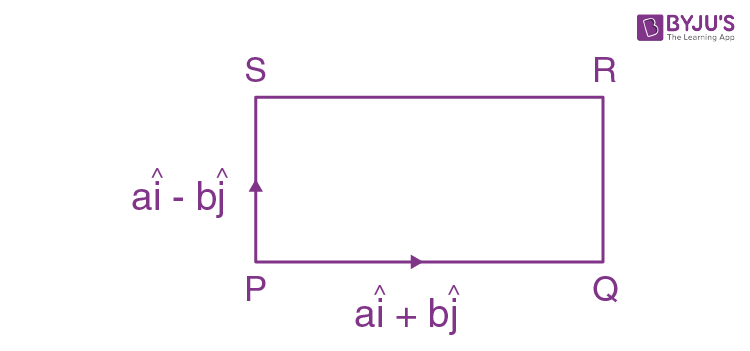Given,

$$\vec{PQ} = a \hat{i} + b \hat{j}$$

$$\vec{PS} = a \hat{i} - b \hat{j}$$
and

$$\vec{w} = \hat{i} + \hat{j}$$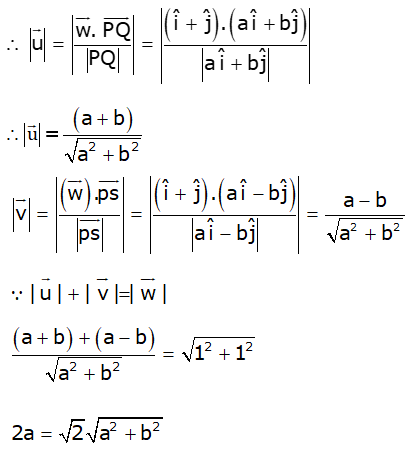Squaring both sides,

4a2 = 2(a2 + b2)

2a2 = 2b2

a = b

Now, area of parallelogram is

$$\begin{vmatrix} \hat {i} &\hat {j} &\hat {k} \\ a &b &0 \\ a &-b &0 \end{vmatrix}$$

⇒ 8 = |-2ab k|

ab = 4 ⇒ a2 = 4 (Because a = b)

a = 2 = b

a + b = 2 + 2 = 4

a – b = 2 – 2 = 0

Length of diagonal of parallelogram =

$$|(a \hat{i} + b \hat{j} + (a \hat{i} - b \hat{j} |$$

= |2a i|

= 2a = 4

$$\vec{PQ} + \vec{PS} = 2a \hat{i}, 2b \hat{j} \neq \lambda \vec{w}$$

Question 12. For non-negative integers s and r, let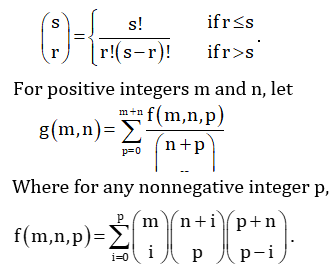Then which of the following statements is/are TRUE?

1. a) (m, n) = (n, m) for all positive integers m, n
2. b) (m, n + 1) = (m + 1, n) for all positive integers m, n
3. c) (2m, 2n) = 2 (m, n) for all positive integers m, n
4. d)(2m, 2n) = ((m, n))2 for all positive integers m, n

Solution:

Solution:

Given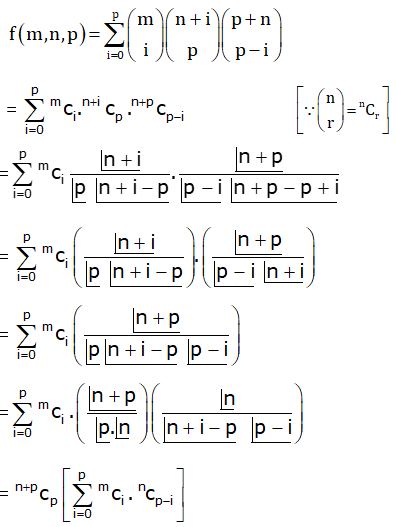= n+pCp [mC0nCp + mC1nCp-1 + …..+ mCmnCp-m ]

Coefficient xp in (1 + x)n (x+1)m

f(m, n, p) = (n+pCp) (m + nCp)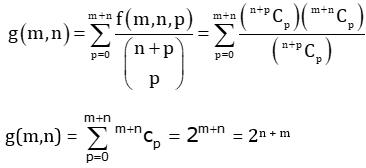g(m, n) = g(n, m)

g(2m, 2n) = 22(m + n) = (2m + n)2 = (g(m, n))2

g(m, n + 1) = 2m + n + 1 = 2(m + 1) + n = g(m+1, n)

Question 13: An engineer is required to visit a factory for exactly four days during the first 15 days of every month and it is mandatory that no two visits take place on consecutive days. Then the number of all possible ways in which such visits to the factory can be made by the engineer during 1-15 June 2021 is_____.

Solution:

Solution:

To select = 4 days

not selected days = 11 days

gaps = 12

12C4 = [12x11x5x9]/24 = 495

Question 14: In a hotel, four rooms are available. Six persons are to be accommodated in these four rooms in such a way that each of these rooms contains at least one person and at most two persons. Then the number of all possible ways in which this can be done is_____.

Solution:

Solution:

By grouping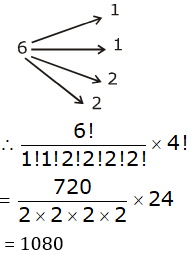Question 15: Two fair dice, each with faces numbered 1, 2, 3, 4, 5 and 6, are rolled together and the sum of the numbers on the faces is observed. This process is repeated till the sum is either a prime number or a perfect square. Suppose the sum turns out to be a perfect square before it turns out to be a prime number. If p is the probability that this perfect square is an odd number, then the value of 14 𝑝 is _____

Solution:

Solution:

Sum is prime =

2 (1,1)

3 (1,2)(2,1)

5 (2,3)(3,2) (1,4) (4,1)

7 (1,6) (2,5) (3,4) (4,3) (5,2) (6,1)

11 (5,6) (6,5)

P(prime) = 15/36 = 5/12

P(perfect square) = 7/36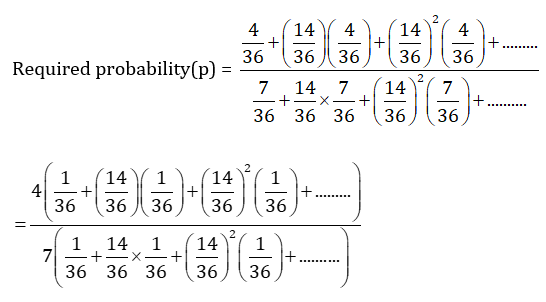p = 4/7

Therefore, 14p = 14 x 4/7 = 8

Question 16: Let the function f: [0, 1] → R be defined by f(x) = 4x/(4x + 2). Then the value of f(1/40) + f(2/40) + f(3/40) + …+ f(39/40) – f(1/2) is _____.

Solution:

Solution:

Given, f: [0, 1] → R

f(x) = 4x/(4x + 2) ..…(1)

Replace x by 1-x

f(1-x) = 41-x/(41-x + 2) = 2/(2+4x) ……(2)

f(x) + f(1-x) = 1 …..(3)

So, f(1/40) + f(2/40) + f(3/40) + …+ f(39/40) – f(1/2) = f(1/40) + f(2/40) + …….+ f(20/40) + …+ f(1 - 3/40) + f(1 – 2/40) + f(1 – 1/40) – f(1/2)

= [f(1/40) + f(1 – 1/40)] + [f(2/40) + f(1 – 2/40)] + [f(3/40) + f(1 – 3/40)] + …..f(20/40) – f(1/2)

From equation (3), we have

= 1 + 1 + 1+ …+1(19 times) + f(20/40) – ½

= 19 + f(1/2) – f(1/2)

= 19

Question 17: Let f:R → R be a differentiable function such that its derivative f’ is continuous and f(π) = -6.

If F: [0, π ] → R is defined by F(x) =

$$\int_0^x f(t) dt$$
, and if
$$\int_0^{pi} (f’(t) + F(x)) cos x dx = 2$$
, Then the value of f(0) is _____

Solution:

Solution:

Given, F: [0, π ] → R

F(x) =

$$\int_0^x f(t) dt$$

Using Newton Leibniz

F’(x) = f(x) d/dx (x) – f(0) d/dx (0)

F’(x) = f(x) …(1)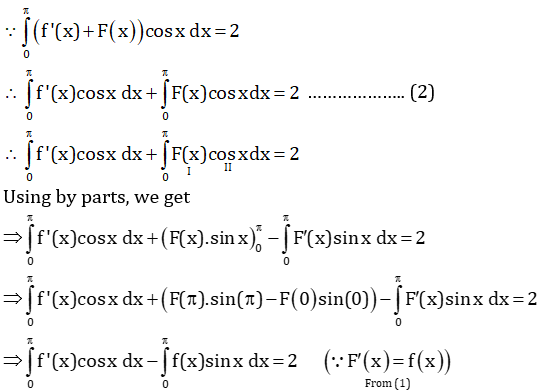$$\int_0^{\pi}$$
(f’(x) cos x - f(x) sin x )dx = 2

$$\int_0^{\pi}\frac{d}{dx}$$
(f(x) cos x)dx = 2

⇒ f(x) cos x|0π = 2

⇒ f(0) = 4

Question 18: Let the function f: (0, π) → R be defined by f(θ) = (sin θ + cos θ)2 + (sinθ - cos θ)4 . Suppose, the function f has a local minimum at θ precisely when θ ∈ {λ1 π, … , λr π}, where 0 < λ1< ⋯ < λr < 1.Then the value of λ1 + ⋯ + λr is _____

Solution:

Solution:

Given, f(θ) = (sin θ + cos θ)2 + (sin θ - cos θ)4

= (sin2 θ + cos2 θ) + 2 sin θ cos θ + ((sin θ - cos θ)2)2

= 1 + sin2 θ + (sin2 θ + cos2 θ - 2sin θ cos θ)2

= 1 + sin2 θ + (1 – sin2 θ)2

= 1 + sin2 θ + 1+ sin2 2 θ – 2sin2 θ

= sin2 2 θ - sin2 θ + 2

⇒ f(θ ) = sin( 2θ - ½)2 + 7/4

Because, θ ∈ [0, π]

Therefore, 2 θ ∈ [0, 2 π]

f(θ) min. when sin 2 θ = ½

Therefore, 2 θ = π/6, 5 π/6

θ = π/12, 5 π/12

λ1 = 1/12 and λ2 = 5/12

So, λ1 + λ2 = ½ = 0.50

### JEE Advanced 2020 Maths Paper 2 Solutions